Free Printable Worksheets Covering Addition and Subtraction with Whole Numbers and Fractions

Find the Missing Addends Worksheet (Set 3)Find the Missing Addends Worksheet (Set 4)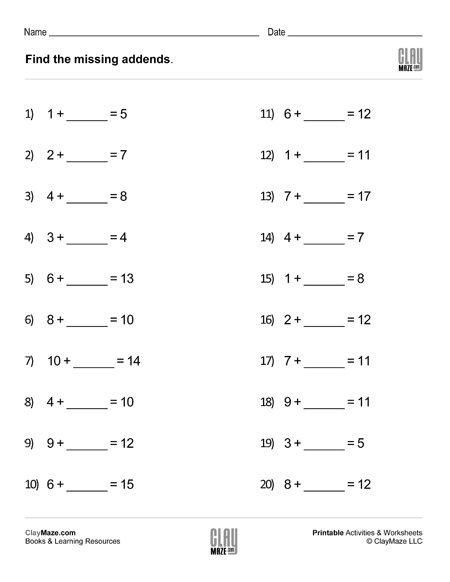Use this worksheet for practice in addition and subtraction facts. – 20 problems...

Word Problems I: Addition & Subtraction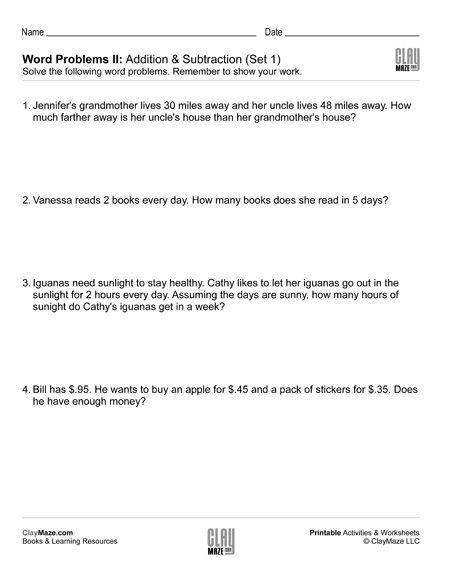These are sets of basic addition and subtraction word problems. The student should read through each problem thoroughly to answer the questions and be sure to show their work below each one. These ...

Word Problems II: Addition & SubtractionThese sets of word problems are slightly more challenging than the Word Problems I sets. The student will need to use addition and subtraction to solve, but some problems use a little bit larger nu...

Time Word Problems: Analog ClocksThese sets of worksheets are great for practice telling time with analog clocks and for understanding how to add and subtract with different time intervals. On these worksheets, the student is aske...

Subtraction Worksheet – 3 Digit Subtraction with Regrouping (Set A)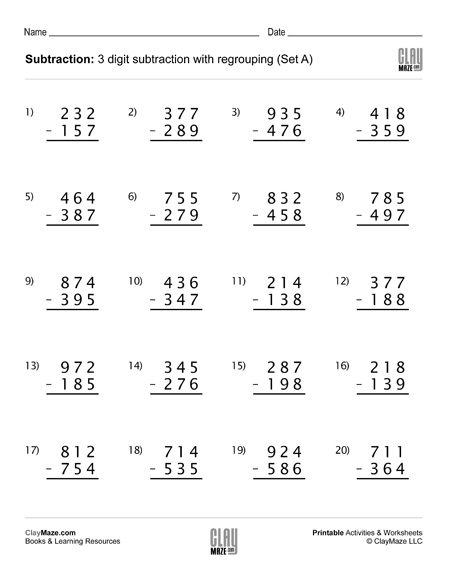This worksheet contains 20 subtraction problems with regrouping. There is extra spacing between the digits for borrowing....

Subtraction Worksheet – 3 Digit Subtraction with Regrouping (Set B)Download this worksheet for subtraction practice with regrouping. The problems use 3 digit numbers with spacing between the digits to allow room for borrowing. There are 20 problems....

Subtraction Worksheet – 3 Digit Subtraction with Regrouping (Set C)Use this 3 digit subtraction worksheet with regrouping for lots of practice. There are 20 problems and numbers have enough spacing between the digits for borrowing....

Subtraction Worksheet – 3 Digit Subtraction with Regrouping (Set D)3 digit subtraction worksheet with regrouping – 20 problems. There is extra spacing between the digits for borrowing....

3 digit addition worksheet with regrouping (Set 1)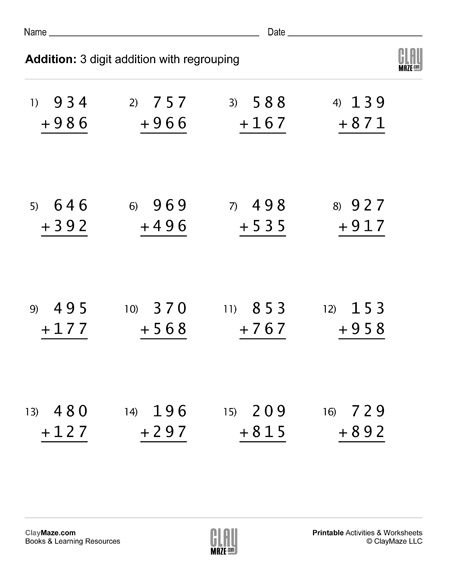3 digit addition worksheet with regrouping (Set 2)3 digit addition worksheet with regrouping (Set 3)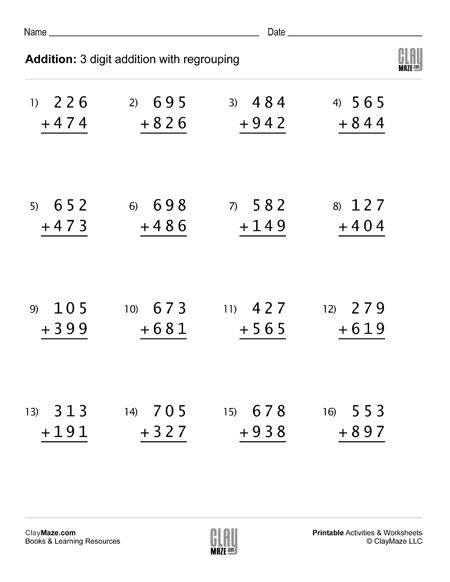This math worksheet contains 16 problems of 3 digit addition with regrouping – good for first grade, second and third grade addition practice....

3 digit addition worksheet with regrouping (Set 4)Free math addition worksheet with 3 digit numbers. All problems in this set are on addition with regrouping....

Build Your Own Math Problems – Fun Paper Cut OutsThis is a great rainy day activity sheet. This math learning project is a little more interactive in that the child gets to cut out the squares necessary to build the problems and then can either a...

Subtraction Worksheet – Fill in the Blanks Subtraction Facts (Set 1)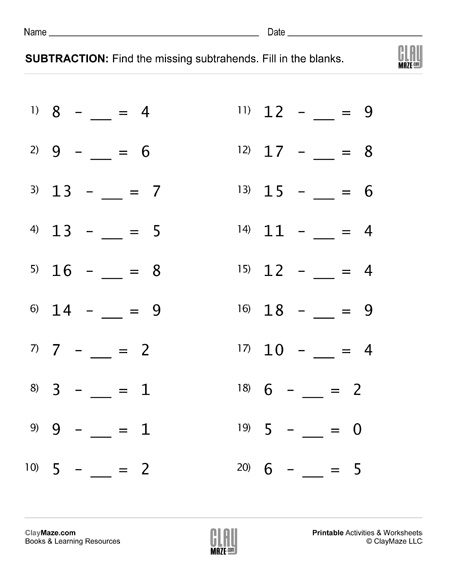Subtraction practice worksheet. Fill in the blanks – Find the missing subtrahend. Solutions included in download....